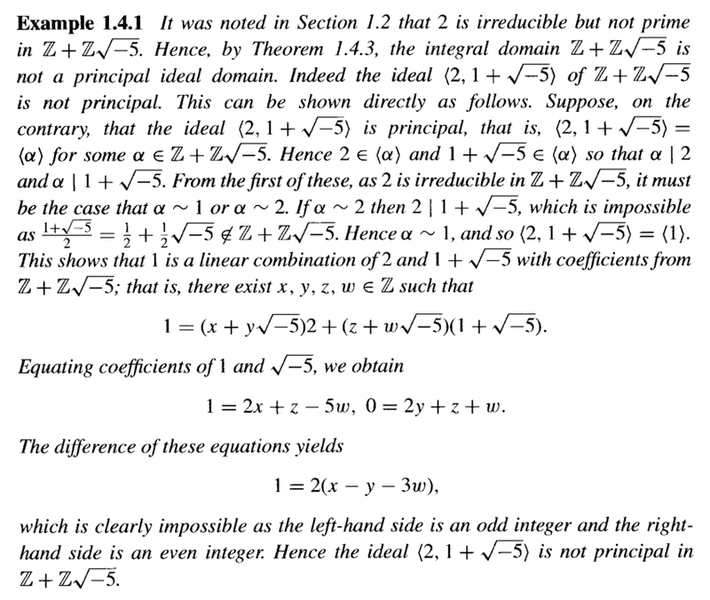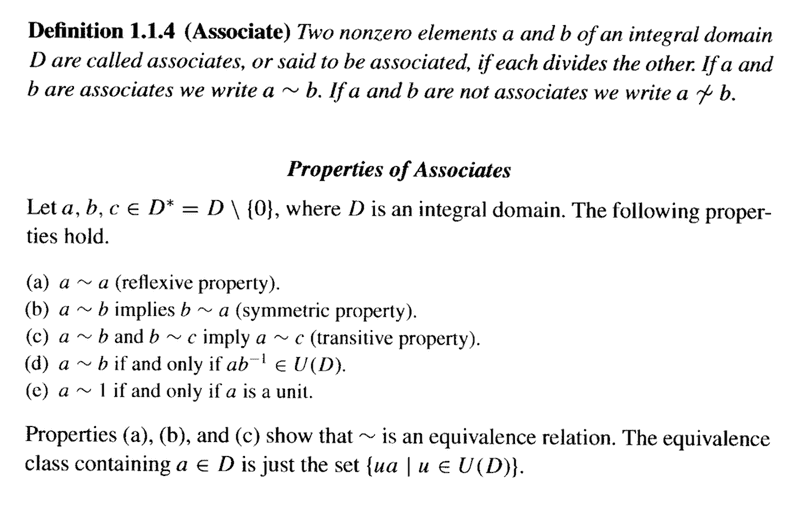# Irreducibles and Primes in Integral Domains ...

Gold Member
I am reading "Introductory Algebraic Number Theory"by Saban Alaca and Kenneth S. Williams ... and am currently focused on Chapter 1: Integral Domains ...

I need some help with understanding Example 1.4.1 ...

Example 1.4.1 reads as follows:In the above text by Alaca and Williams we read the following:

"... ... From the first of these, as ##2## is irreducible in ##\mathbb{Z} + \mathbb{Z} \sqrt{ -5 }##, it must be the case that ##\alpha \sim 1## or ##\alpha \sim 2##. ... ...

My question is as follows ... how does ##2## being irreducible imply that ##\alpha \sim 1## or ##\alpha \sim 2##. ... ...?

Hope someone can help ...

Peter

================================================================================

NOTE

The notation ##\alpha \sim 1## is Alaca and Williams notation for ##\alpha## and ##1## being associates ...

Alaca's and Williams' definition of and properties of associates in an integral domain are as follows:## Answers and Replies

fresh_42
Mentor
My question is as follows ... how does ##2## being irreducible imply that##\alpha \sim 1## or ##\alpha \sim 2##. ... ...?
##\alpha \mid 2## means ## \alpha \cdot q=2## for some ##q##. Now irreducibility of ##2## implies either ##\alpha ## or ##q## is a unit. If ##\alpha ## is a unit, then ##\langle 2, 1+\sqrt{-5} \rangle = \langle \alpha \rangle = \mathbb{Z}+ \mathbb{Z}\sqrt{-5}## which is wrong since it doesn't contain, e.g. ##3##. Now if ##q## is a unit, then ##\alpha = 2 \cdot q^{-1} \mid 1+\sqrt{-5}## which means ##2 \mid 1+\sqrt{-5}## which is also wrong.

It is simply the definitions that are used. You should try to find those things by yourself. It's always a matter of practice. If you will have done it several times, you will start to "see" things more quickly. As a general advice: always try to use what's given, here that ##\alpha \mid 2## and ##2## cannot properly be written as ##2=a\cdot b##. Part of the fun reading those books is to read them as a book of riddles where you're supposed to solve them. A bit like solving crosswords if you like.

•lavinia and Math Amateur
WWGD
Science Advisor
Gold Member
Does every infinite ring (meaning infinite as a set) have infinitely-many primes?

fresh_42
Mentor
Does every infinite ring (meaning infinite as a set) have infinitely-many primes?
I guess you're not satisfied by ##R := (\mathbb{Z}; x\cdot y = 0 \;\forall \; x,y \in \mathbb{Z})## or ##R := \mathbb{R}##.

WWGD
Science Advisor
Gold Member
I guess you're not satisfied by ##R := (\mathbb{Z}; x\cdot y = 0 \;\forall \; x,y \in \mathbb{Z})## or ##R := \mathbb{R}##.
Yes, I would prefer a non-trivial multiplication. Ah, I see, good point with the Reals, I guess we need to EDIT exclude certain types of multiplication actions ( non-transitive?).EDIT 2: Maybe it is more meaningful to work with Euclidean rings here?

fresh_42
Mentor
Yes, I would prefer a non-trivial multiplication. Ah, I see, good point with the Reals, I guess we need to EDIT exclude certain types of multiplication actions ( non-transitive?).EDIT 2: Maybe it is more meaningful to work with Euclidean rings here?
I think fields are already an example. Also matrix rings might do.

But let's see how Euclidean rings work. Since Euclidean rings are principal ideal domains, which are unique factorization domains, and we are only allowed a finite number of primes, all elements have to look like ##\varepsilon \cdot p^{m_1}_1 \ldots p^{m_n}_n## with a unit ##\varepsilon## and ##(m_1 ,\ldots , m_n) \in \mathbb{N}_0^n##. I guess ##n=1## is a reasonable assumption here to start a search for an example. So all elements are of the form ##\varepsilon \cdot p^m## which I think is impossible in an integral domain with unit. (Just scratched a few lines with ##1+1=p^k\, , \,1+1+1=p^l## etc.). Maybe there's an induction argument to rule out the cases ##n>1##, too. And this without using the full advantages of a Euclidean ring. So my guess is, it's impossible, but I don't have a slim argument at hand.

•WWGD
fresh_42
Mentor
How about the usual proof that there are inifnitely many primes: Multiply all (if finite), add ##1## and all primes are units. This leaves us with rings without any primes, e.g. fields.